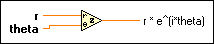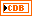# Polar To Complex Function

## LabVIEW 2018 Help

Edition Date: March 2018
Part Number: 371361R-01
View Product Info

### DOWNLOAD (Windows Only)LabVIEW 2016 HelpLabVIEW 2017 HelpLabVIEW 2018 HelpLabVIEW 2019 HelpLabVIEW 2020 Help

Owning Palette: Complex Functions

Requires: Base Development System

Creates a complex number from two values in polar notation.

The dimensions of two matrices that you want to combine must be the same. Otherwise, this function returns an empty complex matrix. The connector pane displays the default data types for this polymorphic function.r can be a scalar number, an array or cluster of numbers, array of clusters of numbers, and so on.theta can be a scalar number, an array or cluster of numbers, array of clusters of numbers, and so on. theta is in radians.r * e^(i*theta) is of the same data type structure as r and theta, with complex representation instead of scalar.

## Polar To Complex Details

Given z in rectangular form z = a + bi, this function converts the polar components according to the following equation:

z = r*cos(theta) + i r*sin(theta)

When you wire matrix data as an input to this function, a VI that includes subVIs that work with the matrix data type replaces the function. The resulting VI has the same icon but contains a matrix-specific algorithm. The node remains a VI if you disconnect the matrix from the input(s). Wire other data types as inputs to restore the original function. If you wire a data type to a function and that data type causes a basic math operation to fail, the function returns an empty matrix or NaN.

Not Helpful